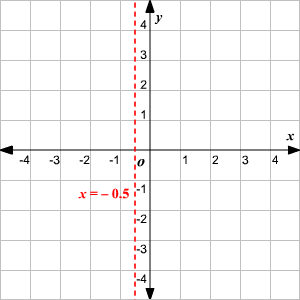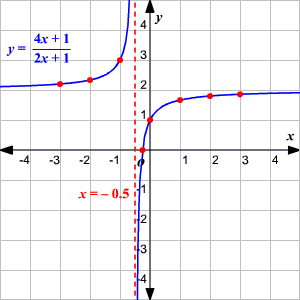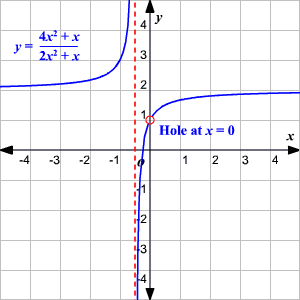# Graphing Rational Functions

Rational functions are of the form $y=f\left(x\right)$ , where $f\left(x\right)$ is a rational expression .

Some of the examples of rational functions are:

$y=\frac{1}{x}$ , $y=\frac{x}{{x}^{2}\text{\hspace{0.17em}}-\text{\hspace{0.17em}}1}$ , $y=\frac{3}{{x}^{4}\text{\hspace{0.17em}}+\text{\hspace{0.17em}}2x\text{\hspace{0.17em}}+\text{\hspace{0.17em}}5}$

The graphs of the rational functions can be difficult to draw. To sketch a graph of a rational function, you can start by finding the asymptotes and intercepts.

Steps involved in graphing rational functions:

1. Find the asymptotes of the rational function, if any.
2. Draw the asymptotes as dotted lines.
3. Find the $x$ -intercept (s) and $y$ -intercept of the rational function, if any.
4. Find the values of $y$ for several different values of $x$ .
5. Plot the points and draw a smooth curve to connect the points. Make sure that the graph does not cross the vertical asymptotes.

Example:

Graph the rational function

$y=\frac{4x\text{\hspace{0.17em}}+\text{\hspace{0.17em}}1}{2x\text{\hspace{0.17em}}+\text{\hspace{0.17em}}1}$

The vertical asymptote of a rational function is $x$ -value where the denominator of the function is zero. Equate the denominator to zero and find the value of $x$ .

$\begin{array}{l}2x+1=0\\ x=-\frac{1}{2}\end{array}$

The vertical asymptote of the rational function is $x=-0.5$ .This function has the $x$ -intercept at $\left(-\frac{1}{4},0\right)$ and $y$ -intercept at $\left(0,1\right)$ . Find more points on the function and graph the function.Sometimes the given rational function has to be simplified, before graphing it. In that case, if there are any excluded values (where the function is not defined) other than at asymptotes, then there is additional step involved in graphing the function.

To represent the undefined function, make sure that the function is not continuous smooth curve at the excluded value. This excluded value is usually referred to as hole in the rational function.

For example, the rational function $y=\frac{4{x}^{2}\text{\hspace{0.17em}}+\text{\hspace{0.17em}}x}{2{x}^{2}\text{\hspace{0.17em}}+\text{\hspace{0.17em}}x}$ has a hole at $x=0$ .Please note that the graphs of the rational functions satisfy the vertical line test .